Previous Article                                                                         Next Article

In this article we will continue our discussion of Platonic solids by moving into the topic of spherical systems.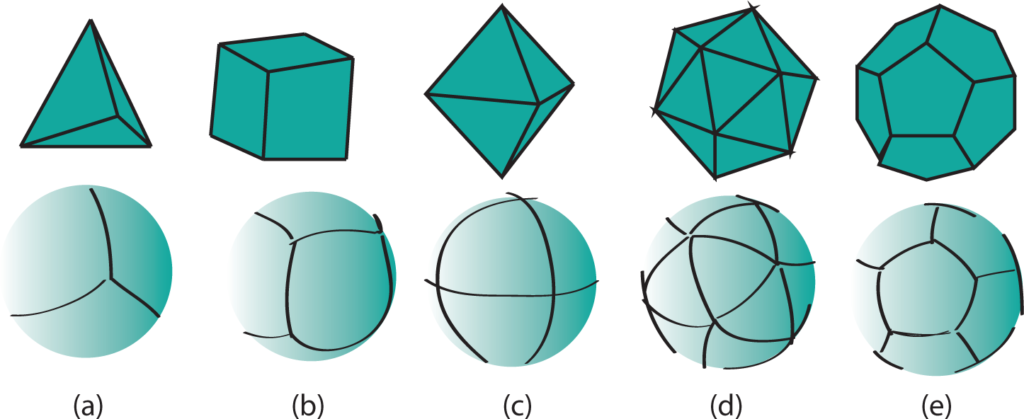Platonic Solids – Close-packed spheres

Each Platonic solid can be built by close-packing different numbers of spheres.

The tetrahedron is composed of 4 spheres.  This is the greatest number that can be in simultaneous contact.  This is the fundamental Platonic solid.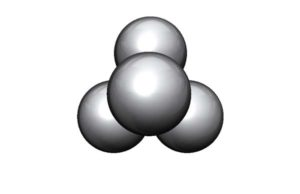The Star Tetrahedron – The tetrahedron and its dual – 8 spheres in all.  A second set of spheres is introduced into the interstices.  This is a compound Platonic solid.  It is also called a “stellated octahedron”.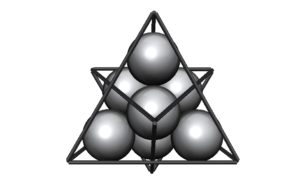The octahedron is composed of 6 spheres.  This is the next most regular grouping of spheres.  Each sphere touches four others in this Platonic solid.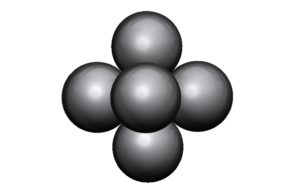The cube is composed of 14 spheres.   A second set of 8 spheres has been introduced into the interstices.  The cube emerges as the dual of the octahedron.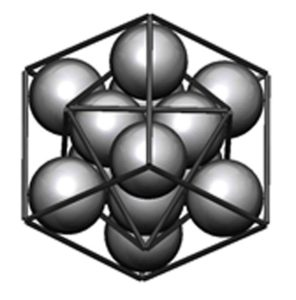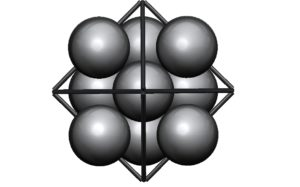Note: The image below is NOT close-packing of spheres.  Close-packing spheres means that each sphere fits within the spaces left by the other spheres.  These spheres are stacked, not close-packed.  They do however form a cube.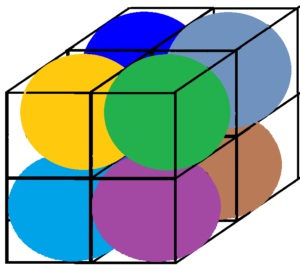The Cuboctahedron – 13 spheres – The closest packing of spheres around a nucleus of equal size gives the cuboctahedron (vector equilibrium).  Each sphere touches the nucleus and 4 others.  This is an Archimedean solid and the balance point between the cube and octahedron.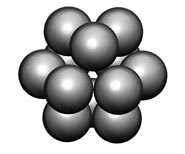The icosahedron is composed of 12 spheres.  This grouping without the nucleus (as in the cuboctahedron) closes into the triangulation of the icosahedral grouping.  Each sphere touches five others.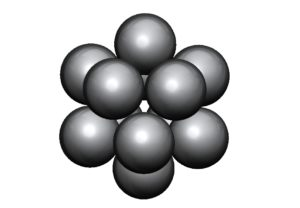The Icosahedron – 32 spheres – A sphere has been introduced into each interstice in this more complex version.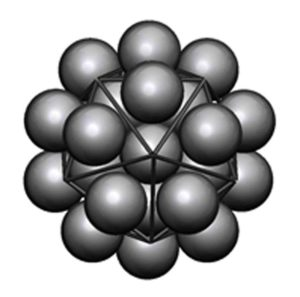The dodecahedron is composed of 32 spheres.  The dodecahedron is the dual of the icosahedron.  The dodecahedron can be seen here.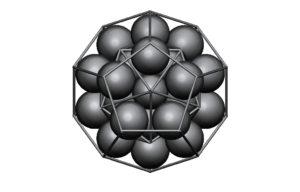This is beautifully illustrated in Keith Critchlow’s excellent book Order in Space: A Design Source Book.  It is also illustrated on the website D’Source at: http://www.dsource.in/course/geometry-design/concepts-3-dimensional

We see from the above that four equal spheres are the greatest number that can be in simultaneous contact.  This produces the tetrahedron.

Six equal spheres are the next regular pattern, with each sphere touching 4 others.  This produces the octahedron.

Twelve equal spheres may surround and touch a nucleus sphere of equal size.  This produces the cuboctahedron.

If you remove the nucleus sphere (the 13th sphere) and push the 12 spheres together you get the icosahedron.

Introducing additional spheres into the interstices generates the dual solid of each.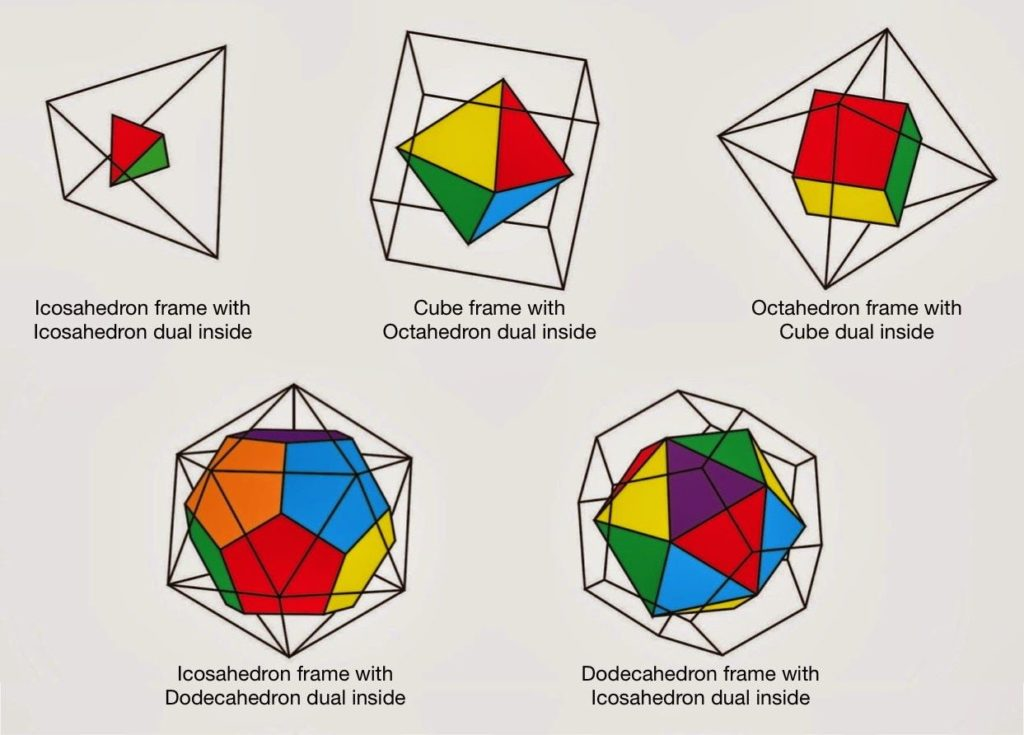The Five Platonic solids and their duals.

The Platonic Solids from the Flower of Life

Here we will discuss the spherical process of how the five Platonic solids are formed from the Flower of Life.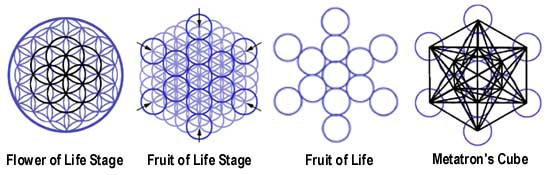The commonly known Flower of Life is shown above in its extended form.  That is, the arcs of circles in the Flower of Life (on far left) that lay on the outermost portion of the design have been extended.

From the extended Flower of Life the Fruit of Life is extracted.  The Fruit of Life consists of 13 circles.

When the centers of the 13 circles are connected you get the image of Metatron’s Cube.  From this all the Platonic Solids can be derived.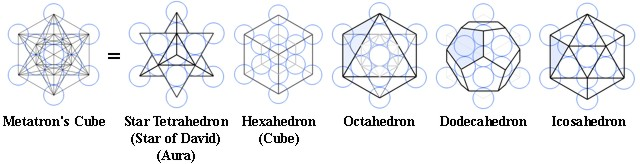Platonic Solids – Planes of Symmetry

Planes of symmetry divide the solids into mirror image halves.

The tetrahedron has               6 mirror planes.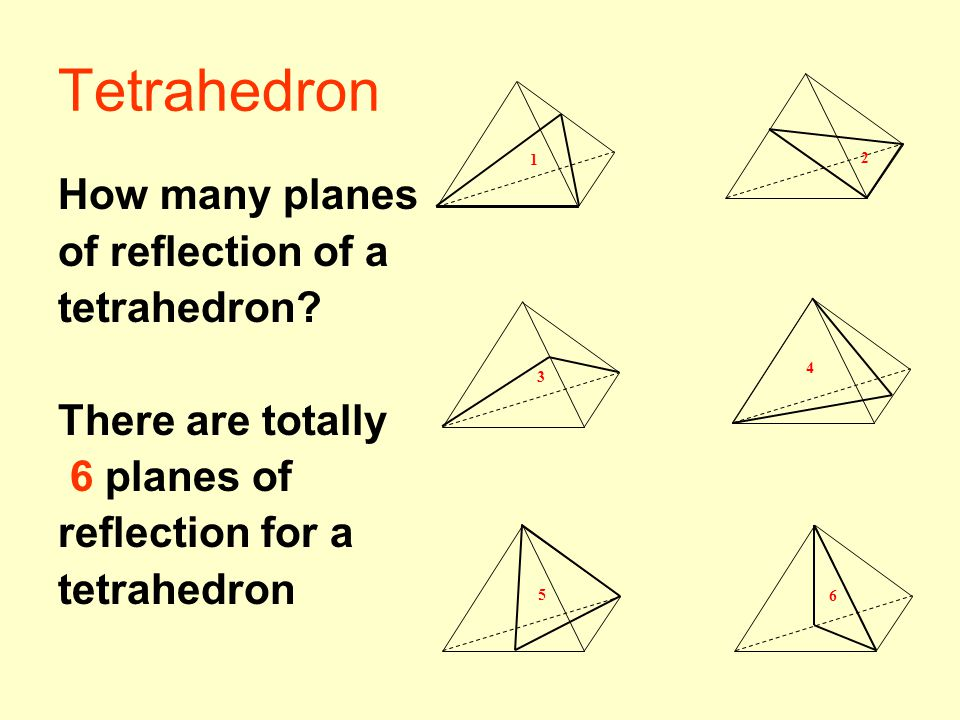The octahedron has                9 mirror planes.

The cube has                           9 mirror planes.

The icosahedron has              15 mirror planes.

The dodecahedron has           15 mirror planes.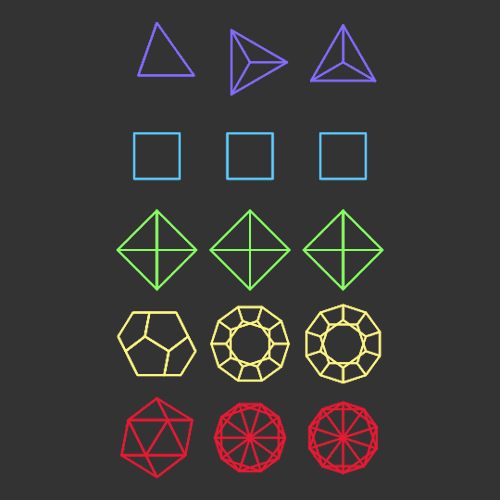Mirror symmetry – involves the exact reflection of a pattern on either side of a ‘mirror line’.        For example: the letter “M”

Rotational symmetry – specifies that a configuration can be rotated some fraction of 360 degrees without changing the pattern.  For example: the letter “S” looks the same after a 180° turn.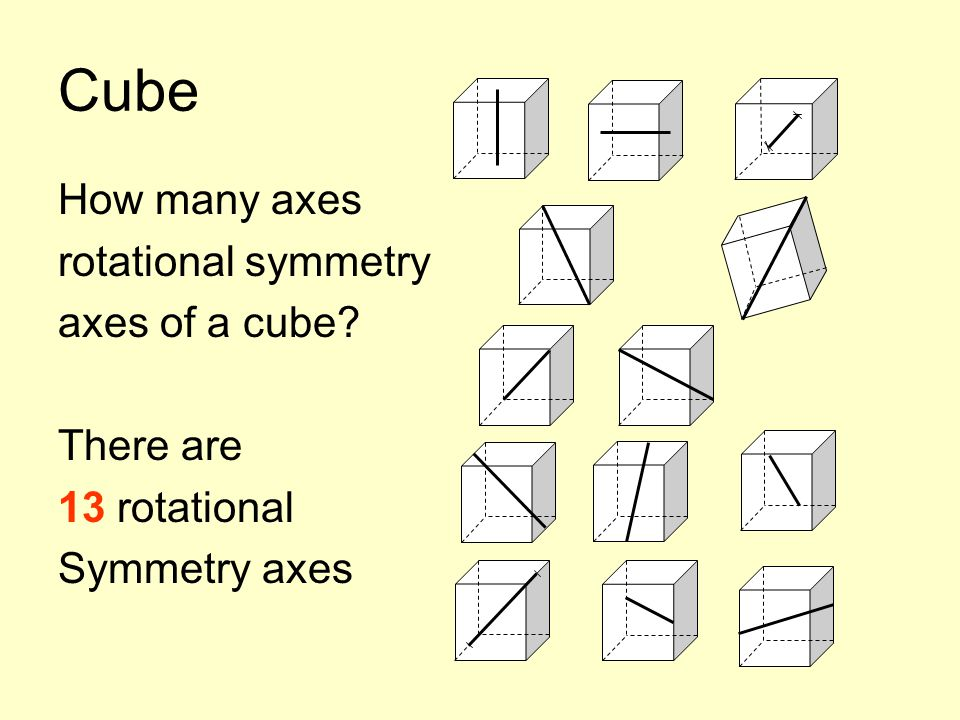In x-fold rotational symmetry, constituents of a pattern are repeated x times about a common center.

When the tetrahedron, octahedron and icosahedron are constructed from Plato’s triangular atoms, paths are defined which make their mirror planes explicit.

Triangles and the Platonic Solids (Plato’s triangular atoms)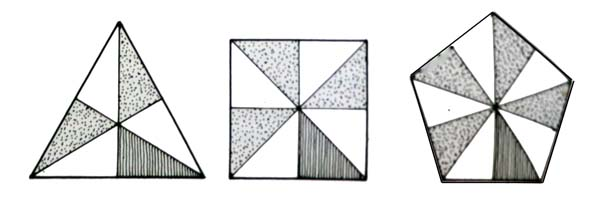Three basic triangles are required to fabricate these five solids:

The scalene half-equilateral triangle: 90°-60°-30°

Makes the tetrahedron, octahedron, and icosahedron.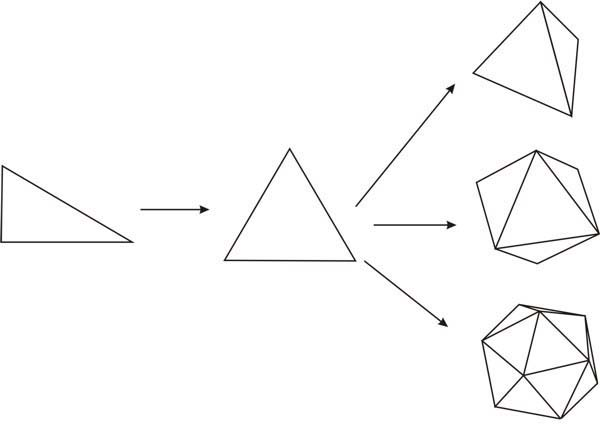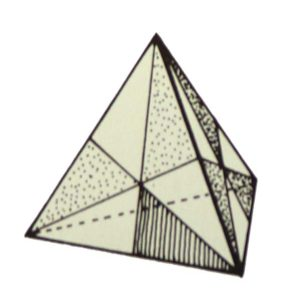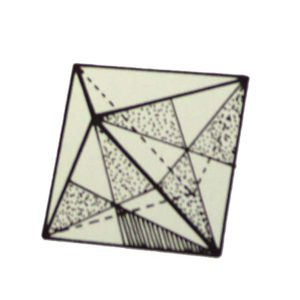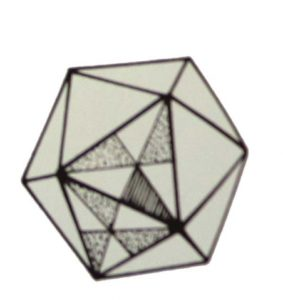The isosceles half square: 90°-45°-45°

Makes the cube.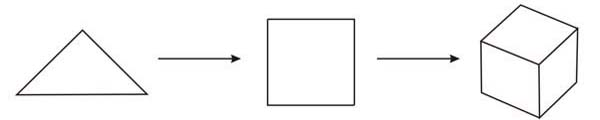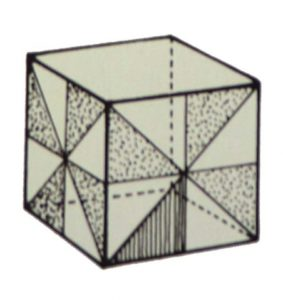The scalene one-tenth of a regular pentagon: 90°54°-36° (see in red below)

Makes the dodecahedron.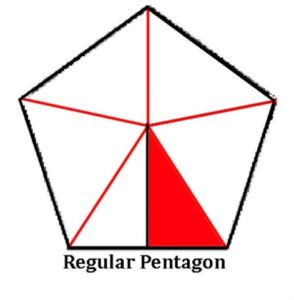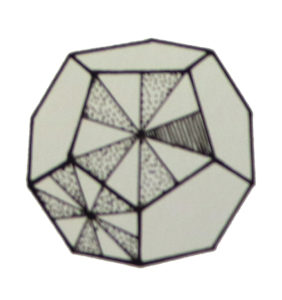See pages 166-167 in Keith Critchlow’s Time Stands Still.

Platonic Solid – Spherical System

Projecting the subdivided (by mirror planes) Platonic Solids onto their circumspheres produces three spherical systems of symmetry.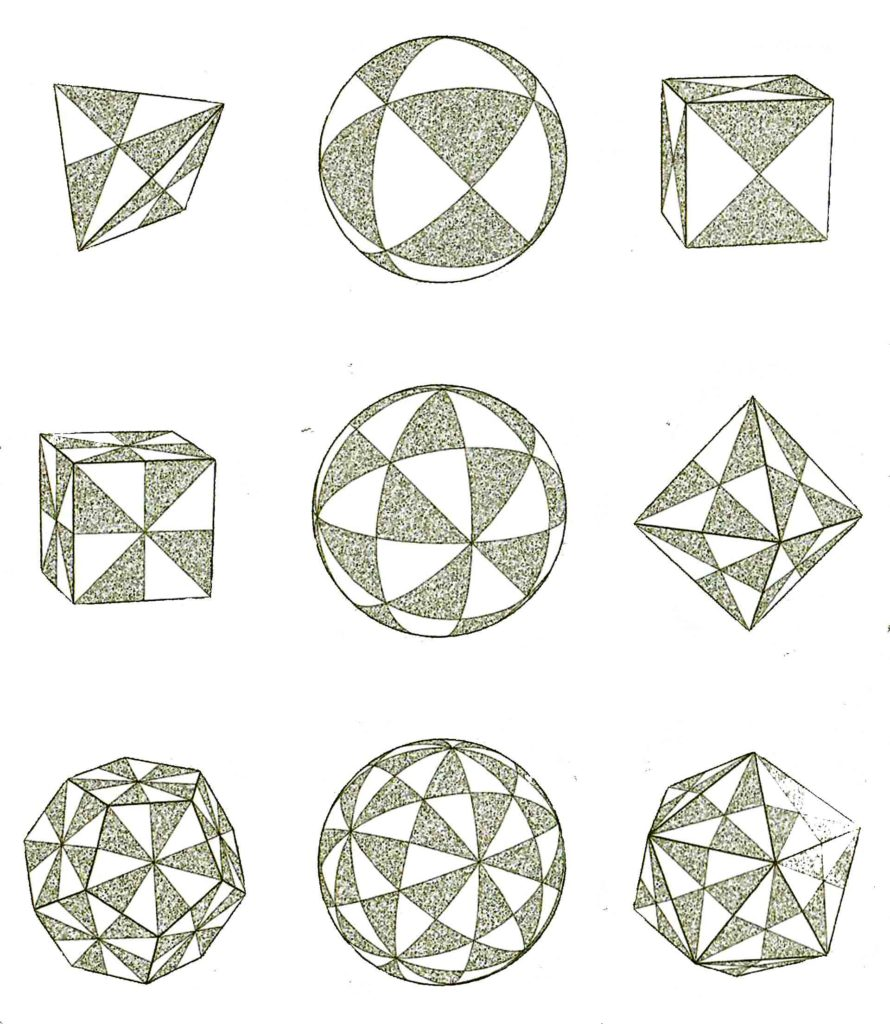Credit: page 149 in the Quadrivium

Each is defined by:

• A spherical triangle with one right angle and:
• one angle of 1/3 a half turn.
• The third angles of the spherical triangles vary from:
• 1/3 a half turn (1/6 a sphere cut in longitudinal slices) (top row above)
• ¼ a half turn (1/8 a sphere cut in longitudinal slices) (middle row above)
• 1/5 a half turn (1/10 a sphere cut in longitudinal slices) (lower row above)

The sequence 1/3, ¼, 1/5 inverts the Pythagorean number triplet 3, 4, 5.

If a spherical surface is divided into the greatest number of equal triangles the result is 120.

The bold triangle below divides a sphere into 20 equal areas.  This creates the spherical icosahedron.

The bold pentagon below divides the sphere’s surface into 12 equal areas.  This creates the spherical dodecahedron.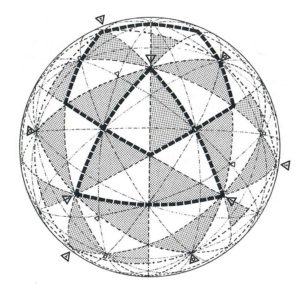Credit: page 173 in Time Stands Still by Keith Critchlow

As Keith Critchlow states, “The study of the heavens is, after all a spherical activity, needing an understanding of spherical coordinates.”1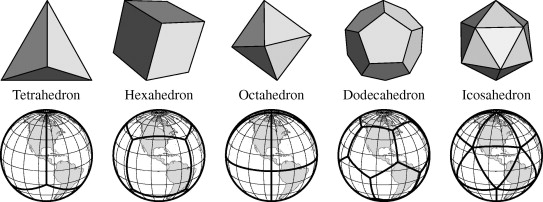Spherical Geometry of Platonic Solids

The image below shows the spherical equivalents of the five Platonic solids.The image below has three rows.

The first row shows the spherical transition of the tetrahedron into its dual the reverse tetrahedron.

The second row shows the spherical transition of the cube into its dual the octahedron.

The third row shows the spherical transition of the dodecahedron into its dual the icosahedron.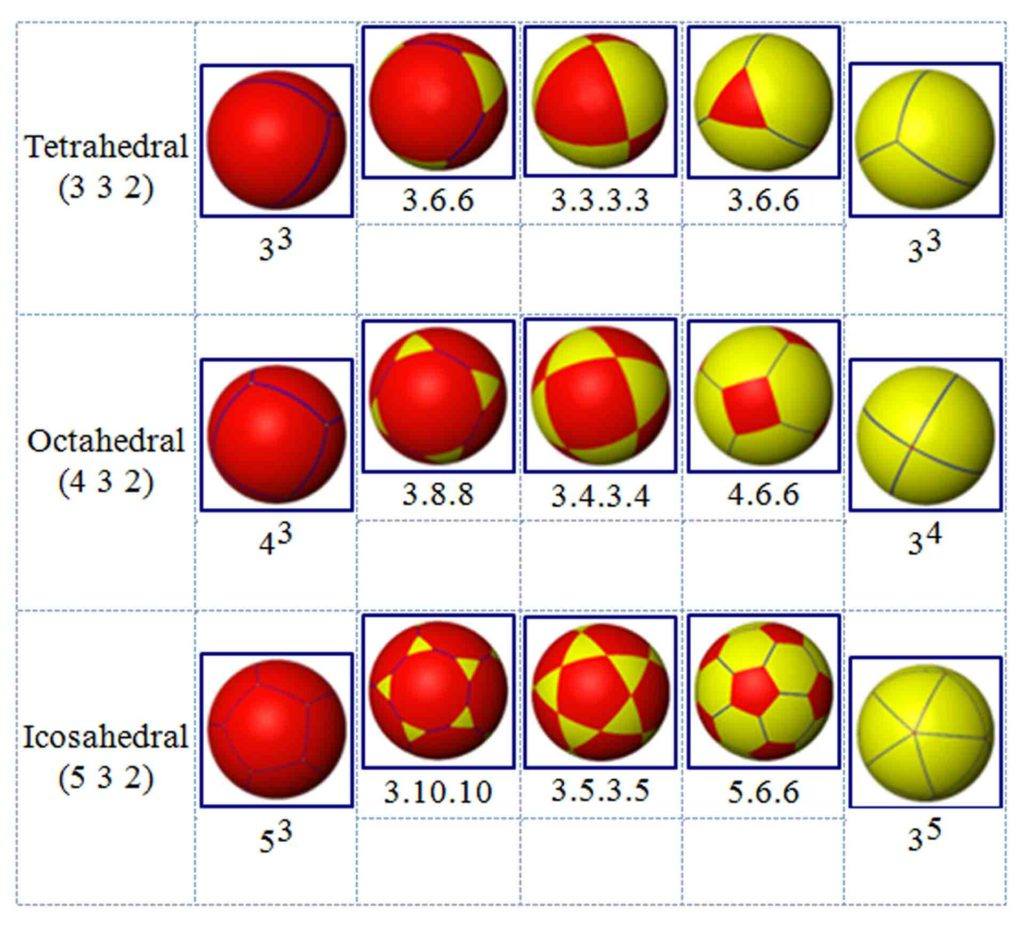“On the sphere, the divisions of symmetry are defined mathematically as ‘great’ circles when their centers coincide with the center of the sphere and describe the largest possible orbit on the sphere’s surface, that is, the circumference.

Small circles are all the remaining circles that can be inscribed on the sphere.  As these are theoretically indefinite in number we can shorten the list by taking those which occur symmetrically and regularly around the whole surface.”2

Greater and Lesser Circles

Arc of a Great Circle – the shortest distance between two points on a sphere’s surface

Lesser Circle – a spherical circle smaller than a great circle, also called a ‘small’ circle.  There are infinitely many of these.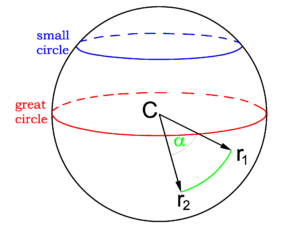Radial Projection – occurs when a polyhedron’s edges are projected onto its circumsphere.

This results in a set of great circle arcs.  These create the spherical versions of the Platonic solids.  We saw examples of this above.  To repeat: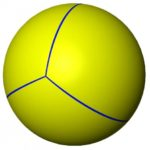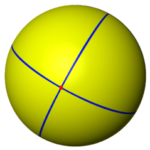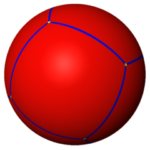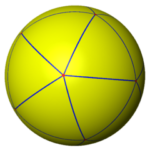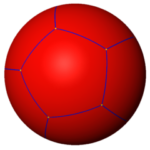The spherical tetrahedron, octahedron, cube, icosahedron & dodecahedron

Platonic Solid Lesser Circles

When set in the same sphere:

• The lesser circles around the dodecahedron’s faces are equal to the lesser circles around the icosahedron’s faces.
• The lesser circles around the cube’s faces are equal to the circles around the octahedron’s faces.

• Shrink the lesser circles in the middle column until they touch each other to define five spherical curiosities seen in the right column.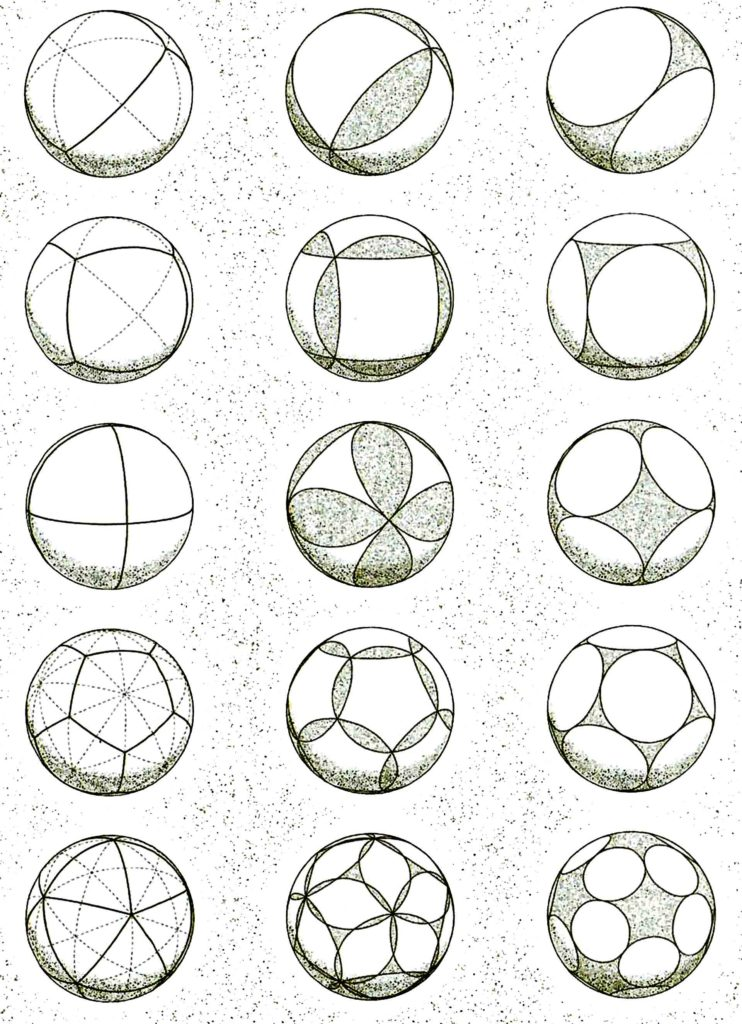Credit: page 151 in the Quadrivium.

Stereographic Projection of Platonic Solids:

“In geometry, the stereographic projection is a particular mapping (function) that projects a sphere onto a plane.  The projection is defined on the entire sphere, except at one point: the projection point.”2

The spherical representation of each Platonic solid is then projected onto a flat surface to create the following patterns.

Here we see the:

• Tetrahedron – 1st row
• Cube – 2nd row
• Octahedron – 3rd row
• Dodecahedron – 4th row
• Icosahedron – 5th row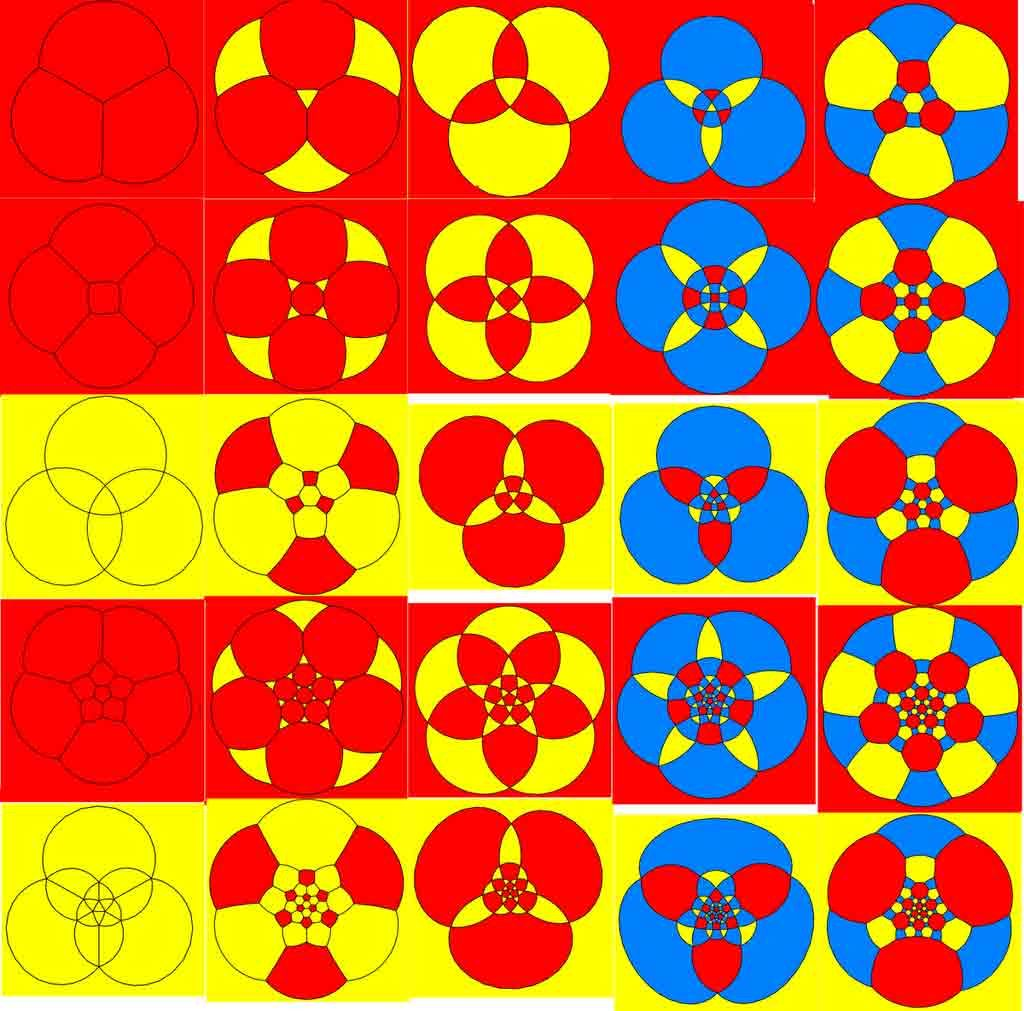Stereographic Projection of a latitude/longitude Grid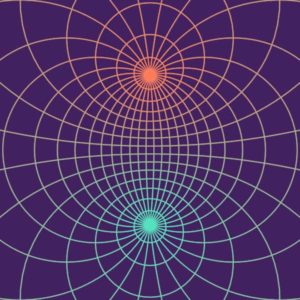Stereographic Projection of a Flat Torus4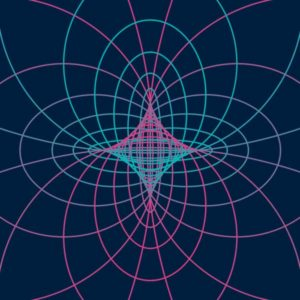1. Critchlow, Keith, Time Stands Still, Brecourt Academic, 2nd edition, 2007
2. ibid.
3. https://en.wikipedia.org/wiki/Stereographic_projection
4. http://community.wolfram.com/groups/-/m/t/1208385